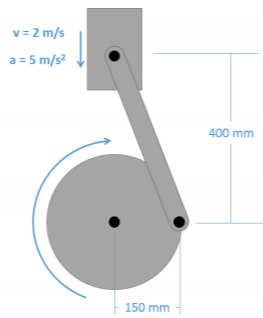# 11.6: Chapter 11 Homework Problems

$$\newcommand{\vecs}{\overset { \rightharpoonup} {\mathbf{#1}} }$$ $$\newcommand{\vecd}{\overset{-\!-\!\rightharpoonup}{\vphantom{a}\smash {#1}}}$$$$\newcommand{\id}{\mathrm{id}}$$ $$\newcommand{\Span}{\mathrm{span}}$$ $$\newcommand{\kernel}{\mathrm{null}\,}$$ $$\newcommand{\range}{\mathrm{range}\,}$$ $$\newcommand{\RealPart}{\mathrm{Re}}$$ $$\newcommand{\ImaginaryPart}{\mathrm{Im}}$$ $$\newcommand{\Argument}{\mathrm{Arg}}$$ $$\newcommand{\norm}{\| #1 \|}$$ $$\newcommand{\inner}{\langle #1, #2 \rangle}$$ $$\newcommand{\Span}{\mathrm{span}}$$ $$\newcommand{\id}{\mathrm{id}}$$ $$\newcommand{\Span}{\mathrm{span}}$$ $$\newcommand{\kernel}{\mathrm{null}\,}$$ $$\newcommand{\range}{\mathrm{range}\,}$$ $$\newcommand{\RealPart}{\mathrm{Re}}$$ $$\newcommand{\ImaginaryPart}{\mathrm{Im}}$$ $$\newcommand{\Argument}{\mathrm{Arg}}$$ $$\newcommand{\norm}{\| #1 \|}$$ $$\newcommand{\inner}{\langle #1, #2 \rangle}$$ $$\newcommand{\Span}{\mathrm{span}}$$$$\newcommand{\AA}{\unicode[.8,0]{x212B}}$$

Exercise $$\PageIndex{1}$$

You are designing a bench grinder with an operating speed of 3600 rpm.

• If you want the grinder to reach its full operating speed in 4 seconds, what must the rate of angular acceleration be in radians per second squared?
• If the grinding wheel has a diameter of 8 inches, what will the speed of the surface of the wheel be?Figure $$\PageIndex{1}$$: A bench grinder.
Solution

$$\alpha = 94.25 \ \frac{rad}{s}$$

$$v = 125.67 \ ft/s$$

Exercise $$\PageIndex{2}$$

A belt-driven system has an input at pulley A, which drives pulley B, which is attached with a solid shaft to pulley C, which drives pulley D. If the input is rotating at 60 rad/s counterclockwise, determine the angular velocity and direction of rotation for the output at D.Figure $$\PageIndex{2}$$: problem diagram for Exercise $$\PageIndex{2}$$. A four-pulley system in which A and B are attached by a belt, C and D are attached by another belt, and B and C are mounted on the same shaft.
Solution

$$\omega_D = 300 \ \frac{rad}{s}$$ counterclockwise

Exercise $$\PageIndex{3}$$

The piston in a piston and crank mechanism has the velocity and acceleration shown below. Using absolute motion analysis, determine the current angular velocity and angular acceleration for the crank.Figure $$\PageIndex{3}$$: problem diagram for Exercise $$\PageIndex{3}$$. A piston descends, causing the crank mounted on a fixed axle below it to rotate due to the bar that connects the piston and the crank.
Solution

$$\omega = 13.33 \ \frac{rad}{s}$$ clockwise

$$\alpha = 100.16 \ \frac{rad}{s^2}$$ clockwise

Exercise $$\PageIndex{4}$$

A trapdoor is being opened with a hydraulic cylinder extending at constant rate of 0.7 m/s. If the door is currently at a twenty-degree angle as shown below, what is the current angular velocity and angular acceleration for the door?Figure $$\PageIndex{4}$$: problem diagram for Exercise $$\PageIndex{4}$$. Side view of a trapdoor being raised by the extension of a hydraulic cylinder that has one end attached to the door and the other fixed to a point on the ground.
Solution

$$\dot{\theta} = 0.896 \ \frac{rad}{s}, \, \ddot{\theta} = -1.246 \ \frac{rad}{s^2}$$

Exercise $$\PageIndex{5}$$

A robotic arm experiences the angular velocities and accelerations shown below. Based on this information, determine the velocity and the acceleration of the end of the arm in the $$x$$ and $$y$$ directions.Figure $$\PageIndex{5}$$: problem diagram for Exercise $$\PageIndex{5}$$. A two-member robotic arm attached to a fixed base experiences rotation from two motors located at the joints.
Solution

$$v_x = 9.44 \ ft/s, \, v_y = 4.39 \ ft/s$$

$$a_x = -33.78 \ ft/s^2, \, a_y = 3.39 \ ft/s^2$$

This page titled 11.6: Chapter 11 Homework Problems is shared under a CC BY-SA 4.0 license and was authored, remixed, and/or curated by Jacob Moore & Contributors (Mechanics Map) via source content that was edited to the style and standards of the LibreTexts platform; a detailed edit history is available upon request.